# Sorting of Queue

## Introduction

Having a good knowledge of Data Structures and Algorithms is a must if your aim is to crack top product-based companies like Facebook, Amazon, Google.

As it is tested rigorously by top companies wherein multiple interview rounds are conducted.

Questions related to Queues are frequently asked in the initial stages of interviews. A Queue is a linear data structure that follows the First-In-First-Out(FIFO) methodology for performing operations. The elements are always inserted to the last of the queue and are removed from the beginning of the queue.

This blog discusses an important interview question, Sorting of Queue

## Sorting of Queue-Problem Statement

The problem statement is, “Given a queue of random elements, you need to sort the queue in ascending order with constant space complexity”

For Example:

As an interviewee, even if you don’t have any idea of the solution, it is recommended to start with the brute force solution, it could be the one that is not optimized at all. In the problem statement, the most simple solution could be:

1. Convert the queue to an Array
2. Sort the array using inbuilt methods
3. Convert the sorted array back to the queue and print it.

The approach will require a linear traversal of the queue making the time complexity O(N) where N is the size of the queue and the space complexity will be O(N) because of the auxiliary array needed to store queue elements.

But the problem statement at hand requires us to solve it in constant space complexity.

Now let us figure out the approach for Sorting of Queue with O(1) space complexity.

## Approach 1: Sorting of Queue

As per the problem statement, the operations allowed on the queue are insertion, deletion, and finding the first element of the queue.

Before moving on to the approach, it is essential to understand the insertion operation in a queue:

As Queue follows FIFO methodology, it can be easily concluded that if an element x is to be inserted into the queue it will be inserted into the rear. Similarly, if another element y is to be inserted into the queue, it will be inserted into the rear i.e. one position right of x.

Refer to the below image for understanding insertion operation in a queue.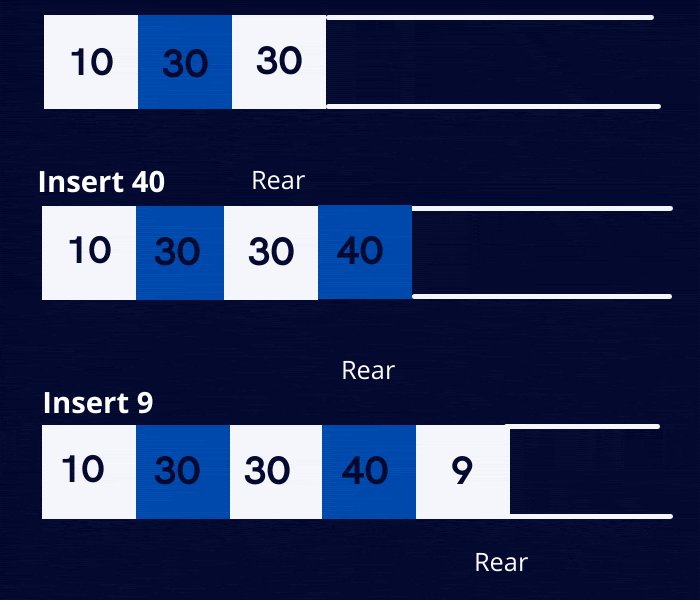And the deletion operation will work as: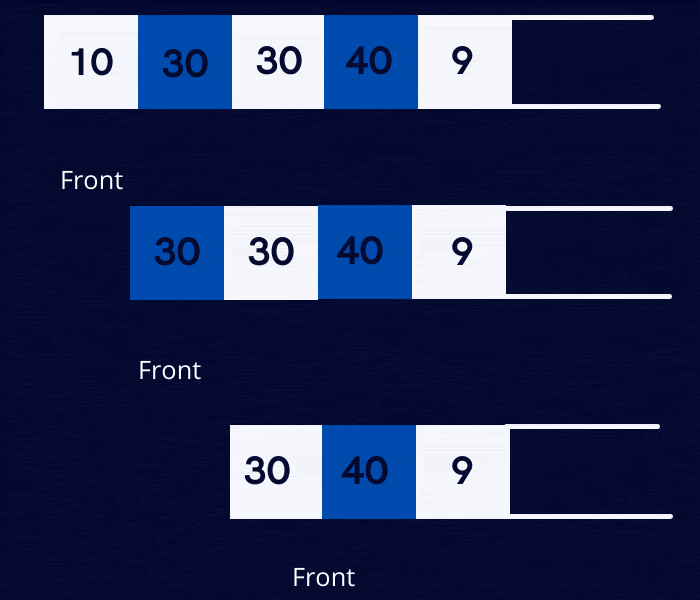If you observe carefully, you will notice that in order to sort the queue, you need to place the minimum element at the rear position followed by the next minimum element, and so on.  Proceeding this way will have the minimum element at the front and the maximum element at the back. Because in each insertion of an element, the remaining elements are shifted one place to the left.

### Algorithm

1. Linearly traverse the queue, in every iteration search for the minimum element, store the index of the minimum element.

2. In the next step insert the element corresponding to the minimum index to the rear of the queue. Now the rearmost element will contain the minimum element of the sequence.

3. Now again traverse the queue, from start to one position behind the rear of the queue. Repeat the above two steps in sequence till the queue becomes sorted.

Refer to the below illustration for a better understanding.

Given an unsorted queue of 6 elements. Iterate over the queue for finding the minimum element.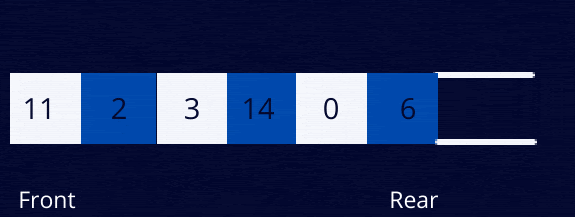The minimum element is 0. This will element will now be shifted to the rear of the queue.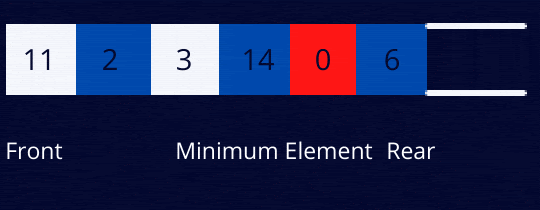0 is now the rearmost element of the queue. The next minimum element is 2, now 2 will be shifted to the right.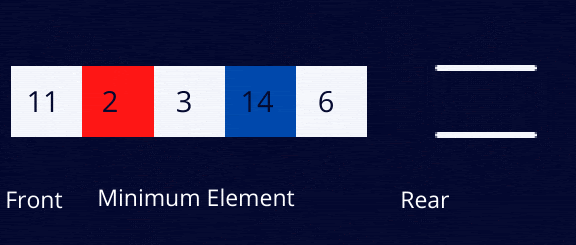2 is now the rearmost element of the queue. The next minimum element is 3, now 2 will be shifted to the right.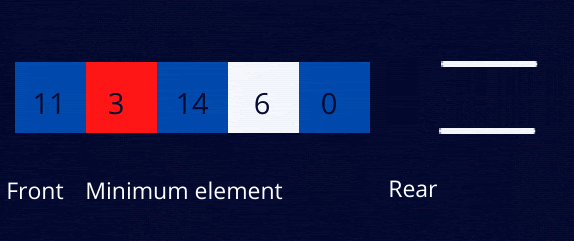3 is now the rearmost element of the queue. The next minimum element is 6, now 6 will be shifted to the right.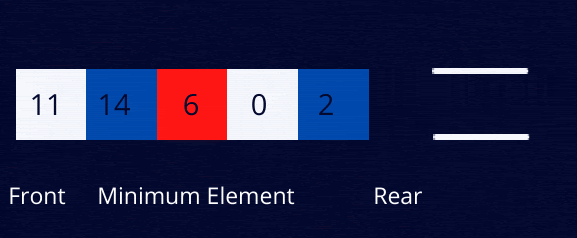6 is now the rearmost element of the queue. The next minimum element is 11, now 11 will be shifted to the right.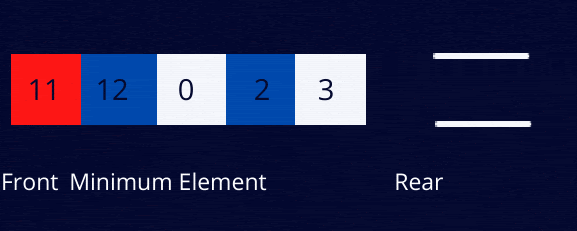11 is now the rearmost element of the queue. The next minimum element is 12, now 12 will be shifted to the right.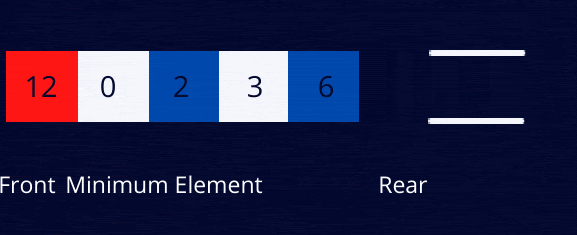The queue is thus sorted now.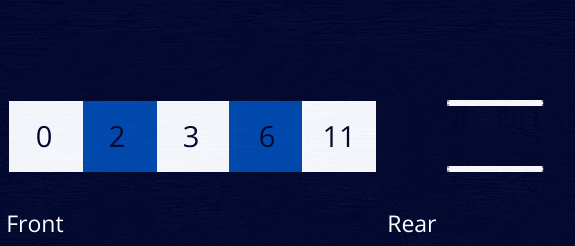The overall problem thus reduces to the following main subproblems:

1. Finding the index of the minimum element in the queue.
In the program below, findIndexOfMinimumElement is used for this purpose.

2. Placing the minimum element at the rear of the queue.
In the program below, insertElementToRear is used for this purpose.

### Implementation

import java.util.Queue;

public class sortQueueWithoutExtraSpace {

// Method to return the minimum element's index in a queue

// The method will check for the minimum element right from

// front of the queue to the index/position specified

// ! In this program, after the position of the index, element are sorted

public static int findIndexOfMinimumElement(Queue<Integer> queue, int index) {

// A general rule is that, if in a sequence minimum element is

// to be found compare each element of the list with the maximum

// possible value and vice versa

int min_index = -1;

int min_element = Integer.MAX_VALUE;

// size() method returns the number of elements in the Queue

int size = queue.size();

for (int i = 0; i < size; i++) {

// peek() method retrieves but does not remove the head of the

int curr_element = queue.peek();

// poll() method retrieves and remove the head of the queue

queue.poll();

if (curr_element <= min_element && i <= index) {

min_index = i;

min_element = curr_element;

}

}

return min_index;

}

// This function will insert the element

// at index position to the rear of the queue

public static void insertElementToRear(Queue<Integer> queue, int index) {

// Currently we don't have access to the element at index position. This

// parameter will store the value at index position as and when accessed in the

// loop

int min_value = 0;

int size = queue.size();

for (int i = 0; i < size; i++) {

// Retrieve but do not remove the element from the front of the queue

int element_at_front = queue.peek();

queue.poll();

if (i != index)

else

min_value = element_at_front;

}

}

// Main function to sort a queue

public static void sortQueue(Queue<Integer> queue) {

for (int i = 0; i <= queue.size(); i++) {

// Find the index of the minimum element from starting of queue to queue.size()

// - i

int index = findIndexOfMinimumElement(queue, queue.size() - i);

// Insert the element at index position to the rear of the queue

insertElementToRear(queue, index);

}

}

public static void main(String[] args) {

// Queue is an interface, we cannot directly create objects of queue

// A class is needed which extends Queue to create an object

int[] arr = { 10, 5, 13, 40, 2 };

// add() method inserts an element to the queue. This method returns true

// upon success and IllegalStateException if no space is currently availab;e

for (int i = 0; i < arr.length; i++) {

}

// Printing the queue

System.out.println("Printing the original queue");

System.out.println(q);

// Call to the sortQueue function. This will sort the queue

sortQueue(q);

// Printing the sorted queue

System.out.println("Printing the sorted queue");

System.out.println(q);

}

}

The output of the above program is:

The time complexity of the above program is O(N^2)

The space complexity is O(1).

1. What is Queue Sorting?
Queue sorting involves the sorting of queue elements in ascending or descending order.
A queue can be sorted using the technique discussed above.

2. What are the applications of Queue Data Structure?
Queue data structure is based on FIFO or First-In-First-Out technique. The applications of the queue data structure are:
(i)Handling of interrupts in real-time systems.
(ii)Serving requests on a single shared resource
(iii)When data is transferred asynchronously between two processes

3. What are the types of queues?
There are four types of queues
(i)Simple Queue
(ii)Circular queues
(iii)Priority Queues
(iv)Double-ended queues

## Key Takeaways

This blog discussed the Sorting of queues with constant space complexity. This is an important interview question. With this done you must try different questions based on the Queue data structure.

Feel free to practice more questions on Codestudio. Codestudio is an excellent platform for practicing questionsguided paths for various technologies, and blogs curated by experts.

“Curiosity is the spark behind the spark of every great idea. The future belongs to the curious”.